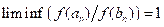# Application of stochastic, statistical and functional methods to analysis of asymptotic behavior of random fields

The project is aimed at studying asymptotic behavior of random fields and functionals related to them, as well as applications of obtained results to the theory of random processes, statistics of random processes, mathematical analysis and mathematical physics. While solving the set of tasks the following results were obtained.

For generalized renewal sets, the strong law of large numbers and the law of iterated logarithm in terms of sets inclusions as well as in metric terms using Hausdorf metrics and Frechet-Nycodim metrics, are obtained. Moreover, functional limit theorem in the sense of convergence in distribution are also provided.
For random signed measures, the uniform strong law of large numbers is considered. Some applications of the obtained results to random measures generated by sums of random variables, marked point processes and stochastic integrals are given.

The class of pseudoregularly varying sequences with nondegenerate groups of regular points is investigated. For such sequences some analogues of properties of correctly pseudoregularly varying functions are obtained. Comparison of properties of pseudoregularly varying functions and sequences are carried out, and certain differences are stated. Moreover, in the work certain classes of functions that generalize pseudoregularly varying functions are studied. Finally, Karamata type integral representations for such functions are given.

For inhomogeneous stochastic differential equation with separation of stochastic and deterministic variables of fairly general type, the asymptotic behavior of solutions in terms of parameters of the equation is obtained. These results are applied to problems on the asymptotic behavior of some specific equations in financial mathematics.

For the given sequencesandand some function f conditions under which the function f preserves equivalence of sequences in the sense of, i.e. conditions under which the relation impliesare studiedThe asymptotic behavior of sums of elements of linear autoregressive sequences in terms of complete convergence and in terms of Hsu-Robbins-Erdos-Spitzer-Baum-Katz series related to it, is investigated. Complete analogues of the Hsu-Robbins-Erdos and Spitzer theorems are obtained, as well as some partial cases of the Baum-Katz theorem. In addition, the Kolmogorov-Marcinkiewicz-Sigmund strong law of large numbers for sums of elements of autoregressive sequence is considered.

An enhanced property of the weak consistency of the Koenker-Bassett estimates in a linear regression model with a nonlinearly transformed Gaussian stationary time series and a singular spectrum as a random noise is obtained.

A new sufficient condition, under which general solutions of the parabolic initial-boundary value problem for the Petrovsky system with homogeneous Cauchy initial conditions are said to be classical, is obtained. This condition is formulated in terms of belonging of the right parts of the problem to some anisotropic Hoermander spaces.

AttachmentSize2020_2105.pdf325.04 KB